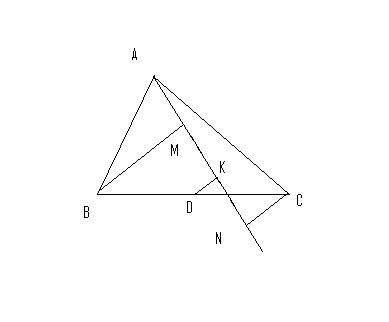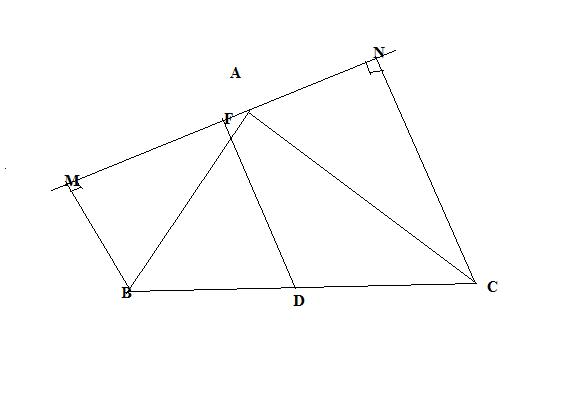# 'g' for geometry

Let AN be any line drawn through A in a Triangle ABC.
Let BM, CN perpendiculars are drawn from B and C to AN and let D be the mid-point of BC, prove that MD = ND.

•rahul ·

can't get ur soln....
either the problem lies with the literature of my question or maybe u r wrong...

here's the exact question with the figure once again......Given a triangle ABC. A line from A meeting BC at O is drawn.
perpendiculars are drawn on AO from B and C at M and N respectively.
If D is the mid point of BC then prove that,
DM = DN

•sougata nag ·let BC & AN meet at O.
now, Î”MOBâ‰ˆÎ”KODâ‰ˆÎ”OCN
MK/BD=KO/OD=ON/OC=KN/CD. as CD=BD so MK=KN so MD=DN.
[proved]

•rahul ·

ya... now that is appreciable...!!

•Shubhodip ·

Denote the intersection of MN and BC by O

since BM || NC

ON/ OC = OM/OB = (ON + OM)/(OC + OB) = MN/BC = MF/BD

so OM/OB = MF/BD = (OM- MF)/(OB - BD) = OF/OD

so OF/OD = MF/BD so the result follows by thales

(No construction, nothing )

•rahul ·

well my soln. using Apollonious..!!The red lines are the contructions i've done..!!

Now, using Appolonius theorem...

In triangle ABC we have,

BM2 + CM2 = 2 (BD2 + DM2) --- (i)

And, in triangle BNC we have,

BN2 + CN2 = 2 (BD2 + DN2) ---- (ii)

on (i) - (ii) we have,

BM2 - BN2 + CM2 - CN2 = 2 (DM2 - DN2)

=> - MN2 + MN2 = 2 (DM2 - DN2)

=> DM = DN

•rahul ·

what's wrong with u??????

In triangle BOM , "D" is not the mid point of OB...!!

How then can u say DF || BM ???????

actually i've already done this question with Apollonius

And here goes the Thales' theorem...and what u r trying to say is...Here, F is the mid point of MN , D is the mid point of BC
then which part of Thales' theorem says DF || BM
rather, if D would have been the mid point of A line through N which would meet
BM at some point then one could say, DF || BM...

nd i don't knw what r u trying to do?????
if u can prove it using thales' theorem by constructing triangles and all then do it....
i just want to see the proof....
that's all....!!

•Shubhodip ·

(Breaking the promise)

F is the mid point of MN

then BM || DF || NC

What the hell is wrong here ?

Can't you just try to prove BM || DF || NC ?

•rahul ·

i think the concept that u r using here is cent % wrong....
nd has no relation with thales' theorem.....
jst look at the statement uv posted..
nd thats wht iv been trying to tell u...

•Shubhodip ·

(for the last time )

Let F be the mid point of MN

DF ||BM || NC (if u cant get this by thales, use complex number/coordinate )

so MN perpendicular to DF, F is midpoint so FD is it's perpendicular bisector ; so DM = DN

•Shubhodip ·

This is extremely easy, it probably reduces to the fact that , in isosceles triangle ABC, C lies on the perpendicular bisector of AB

•Shubhodip ·

F is midpoint of MN, D is mid point of BC

BM || NC

so BM ||NC || DF (use thales)

•Anik Chatterjee ·

@shubhodip couldnt get...can u please explain?
@rahul can u give the solution.. y r images not being uploaded properly?? this is annoying ...

•rahul ·

well i to have a soln..
did it using Apollonius theorem..!!

•rahul ·

what?
how can DF||BM||CN by ur statement?

afterall u can't make the median perpendicular to MN from D unless u prove the statement
either
DM = DN (That's what u need to prove)

or,

that DF bisects angle MDN..

•Shubhodip ·idk if u can see the image, at least I don't

Let F be the midpoint of MN

so DF || BM || CN

so MN perpendicular to DF, F is midpoint so FD is it's perpendicular bisector ; so DM = DN

•rahul ·

@Shubhodip - i don't think so... can u show ur work?

@Anik - try to figure out what the statement says... its easy..!!

•Anik Chatterjee ·

how can CN be perpendicular to AN??

•